System ProgrammingLearn about Pointers in C++

# Learn about Pointers in C++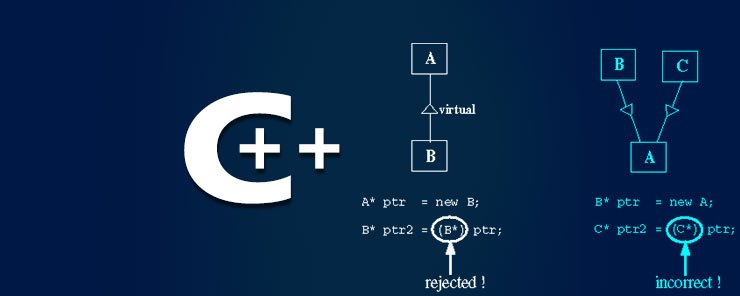Now, grab your coffee, sit in your favourite chair, give me your attention, and bear with me; we are going to talk about Pointers. An advanced feature of C language or C++ language that needs your full concentration. So, are you ready? Let’s go.

What are Pointers?
Pointers are an advanced C++ feature that breaks the limits of the ordinary programming languages by allowing the developer to directly deal with memory. So, Pointers are very powerful, that is true, but they could be very dangerous as well. So, they should be used with care. Some popular languages like Java don’t have Pointers.

Why Pointers?
– If they are not easy to use, could be dangerous, and some powerful languages like Java don’t need them at all, so why should we care about Pointers?!

The answer is simple: the capabilities that Pointers offer are great to the extent that makes it good for a C/C++ learner to know about them.

For instance, Pointers can be used to allow a function to modify one or more variables passed to it as arguments. This is a very useful feature (knowing that a function can return only one value). Pointers also provide another way to access array elements.

So, this article will give you some basic knowledge about Pointers, and at the end of the day, the choice either to count on Pointers or not is yours.

Example
Consider the following program:

```#include <iostream.h>
#include <conio.h>
int main()
{
float *ptr;
*ptr = 10.5;
clrscr();
cout 	<< ptr << endl;
cout	<< *ptr;
getch();
return 0;
}
```

When executed, this code should print the following output: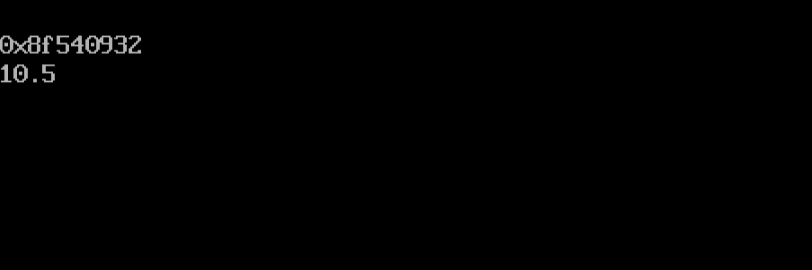The 10.5 is understood, but what the hell is 0x8f540932?

Okay, let’s explain.

• The statement:
`float *ptr;`

This declares a pointer variable to a float value. Notice the usage of the * that means a pointer to. So, ptr itself is a pointer variable whose value is the address of a memory location that stores a float value.

• The statement:
`*ptr = 10.5;`

Assigns the decimal value 10.5 to the memory location whose address is ptr.

• The statement:
`cout << ptr << endl;`

prints the value of the variable ptr, which is the address of the memory location.

• The statement:
`cout   << *ptr;`

prints the contents of the memory location at address ptr.

Have you got the idea?

```ptr is a memory address.
*ptr is its content.```

Using Pointers to Access Array Elements
An array is stored in the memory as a set of consequent memory segments, one for each element. Pointers use this fact to provide another way to access array elements.

Given a normal array of integers:

`int numbers[] = { 48, 56, 67, 73 };`

Have you ever tried (or miss-typed) to print the array itself (without providing index)? If you execute:

`cout << numbers;`

Surprisingly, you will get the following output: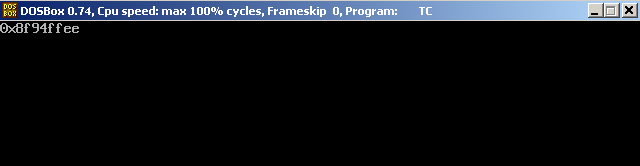Yes, it is! This is simply because “an array name refers to its address in memory”. So, to print the first element in the above array, use the following statement:

`cout << *numbers;`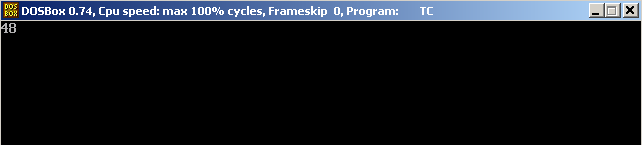To print the second element:

`cout << *(numbers+1);`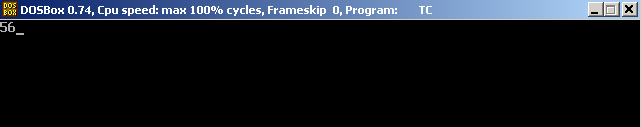Similarly, to print the nth element, use the statement:

`cout << *(numbers+n-1);`Passing Pointers to Functions
Besides to the capability to pass arguments to functions by reference, which allows the function to change the original variables, pointers also can be passed as arguments to functions. This also allows the function to modify the original variables, if needed.

Example
Back to the swap example.

```#include<iostream.h>
#include<conio.h>
void swap(int *arg1, int *arg2)
{
int temp = *arg1;
*arg1 = *arg2;
*arg2 = temp;
}
void main() {
clrscr();
int x=9, y=14;
cout << "\nBefore: x = " << x << " , y = " << y << endl;
swap(&x,&y);
cout << "\nAfter:  x = " << x << " , y = " << y;
getch();
}
```

When executed, the program should swap the contents of the variables x and y.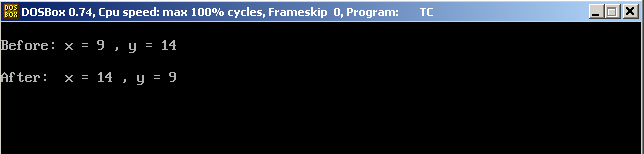Summary

• A Pointer is a variable that stores a memory address.
• To mark a variable as pointer, use the * operator in its declaration.
• A pointer points to a memory address that stores data of specific type.
• To access this data for reading/writing, the pointer variable is preceded with *
• Pointers provide an alternate way to access an array.
• Pointers to variables can be passed as arguments to functions. This gives the function the capability to modify the original variables.

That was an introduction to Pointers. See you in the next article.

### How Challenging Is B2B Marketing?

21,501Fans
4,218Followers
103,000Subscribers

### How Challenging Is B2B Marketing?

- Advertisement -